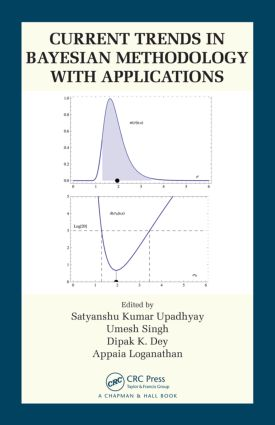# Current Trends in Bayesian Methodology with Applications

## 1st Edition

Chapman and Hall/CRC

680 pages

Paperback: 9780367377625
pub: 2019-07-31
SAVE ~\$14.99
Available for pre-order
\$74.95
\$59.96
x
Hardback: 9781482235111
pub: 2015-05-21
SAVE ~\$33.00
\$165.00
\$132.00
x
eBook (VitalSource) : 9780429172373
pub: 2015-05-21
from \$28.98

FREE Standard Shipping!

### Description

Collecting Bayesian material scattered throughout the literature, Current Trends in Bayesian Methodology with Applications examines the latest methodological and applied aspects of Bayesian statistics. The book covers biostatistics, econometrics, reliability and risk analysis, spatial statistics, image analysis, shape analysis, Bayesian computation, clustering, uncertainty assessment, high-energy astrophysics, neural networking, fuzzy information, objective Bayesian methodologies, empirical Bayes methods, small area estimation, and many more topics.

Each chapter is self-contained and focuses on a Bayesian methodology. It gives an overview of the area, presents theoretical insights, and emphasizes applications through motivating examples.

This book reflects the diversity of Bayesian analysis, from novel Bayesian methodology, such as nonignorable response and factor analysis, to state-of-the-art applications in economics, astrophysics, biomedicine, oceanography, and other areas. It guides readers in using Bayesian techniques for a range of statistical analyses.

Bayesian Inference on the Brain. Forecasting Indian Macroeconomic Variables Using Medium-Scale VAR Models. Comparing Proportions: A Modern Solution to a Classical Problem. Hamiltonian Monte Carlo for Hierarchical Models. On Bayesian Spatio-Temporal Modeling of Oceanographic Climate Characteristics. Sequential Bayesian Inference for Dynamic State Space Model Parameters. Bayesian Active Contours with Affine-Invariant Elastic Shape Prior. Bayesian Semiparametric Longitudinal Data Modeling Using NI Densities. Bayesian Factor Analysis Based on Concentration. Regional Fertility Data Analysis: A Small Area Bayesian Approach. In Search of Optimal Objective Priors for Model Selection and Estimation. Bayesian Variable Selection for Predictively Optimal Regression. Scalable Subspace Clustering with Application to Motion Segmentation. Bayesian Inference for Logistic Regression Models Using Sequential Posterior Simulation. From Risk Analysis to Adversarial Risk Analysis. Symmetric Power Link with Ordinal Response Model. Elastic Prior Shape Models of 3D Objects for Bayesian Image Analysis. Multi-State Models for Disease Natural History. Priors on Hypergraphical Models via Simplicial Complexes. A Bayesian Uncertainty Analysis for Nonignorable Nonresponse. Stochastic Volatility and Realized Stochastic Volatility Models. Monte Carlo Methods and Zero Variance Principle. A Flexible Class of Reduced Rank Spatial Models for Large Non-Gaussian Dataset. A Bayesian Reweighting Technique for Small Area Estimation. Empirical Bayes Methods for the Transformed Gaussian Random Field Model with Additive Measurement Errors. Mixture Kalman Filters and Beyond. Some Aspects of Bayesian Inference in Skewed Mixed Logistic Regression Models. A Bayesian Analysis of the Solar Cycle Using Multiple Proxy Variables. Fuzzy Information, Likelihood, Bayes’ Theorem, and Engineering Application. Bayesian Parallel Computation for Intractable Likelihood Using Griddy-Gibbs Sampler. Index.

Satyanshu K. Upadhyay is a professor and head of the Department of Statistics at Banaras Hindu University.

Umesh Singh is a professor in the Department of Statistics at Banaras Hindu University.

Dipak K. Dey is a distinguished professor in the Department of Statistics at the University of Connecticut.

Appaia Loganathan is a professor in the Department of Statistics at Manonmaniam Sundaranar University.